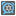• # Morse clock

## Question related to missionMorse Clock

Hello,

In first, sorry for my english.

In morse clock, I have code a program ( you can see in the picture ).

I don't understand why the 2 results are differents.

```def dheure(lim,val):
lst=[8,4,2,1]
heure=''
while lim < len(lst):
if int(val/lst[lim])==1:
heure=heure+'-'
else:
heure=heure+'.'
val=val%lst[lim]
lim+=1

return heure

def checkio(data):
nb='0123456789'
i=0
heure=''
while i < len(data):
cpt=0
if data[i] in nb:
if i == 0:
cpt=2
elif i == 3 or i == 6:
cpt=1
if i == 7:
heure = heure + str(dheure(cpt,int(data[i])))
else:
heure = heure + str(dheure(cpt,int(data[i])))+' '
else:
heure = heure +' : '

i+=1

#replace this for solution
return heure
```